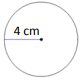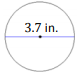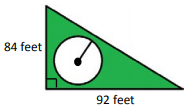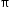# MAFS.7.G.2.4Archived StandardExport Print
Know the formulas for the area and circumference of a circle and use them to solve problems; give an informal derivation of the relationship between the circumference and area of a circle.
General Information
Subject Area: Mathematics
Domain-Subdomain: Geometry
Cluster: Level 2: Basic Application of Skills & Concepts
Cluster: Solve real-life and mathematical problems involving angle measure, area, surface area, and volume. (Additional Cluster) -

Clusters should not be sorted from Major to Supporting and then taught in that order. To do so would strip the coherence of the mathematical ideas and miss the opportunity to enhance the major work of the grade with the supporting clusters.

Date of Last Rating: 02/14
Status: State Board Approved - Archived
Assessed: Yes
Test Item Specifications

• Assessment Limits :

Circles are limited to whole circles and semicircles.

• Calculator :

Yes

• Context :

Allowable

Sample Test Items (4)
• Test Item #: Sample Item 1
• Question:

A circle with its dimensions, in centimeters (cm), is shown.What is the area, in square centimeters, of the circle?

• Difficulty: N/A
• Type: EE: Equation Editor

• Test Item #: Sample Item 2
• Question: A circle with its dimensions, in inches (in.), is shown.What is the area, in square inches, of half the circle?

• Difficulty: N/A
• Type: EE: Equation Editor

• Test Item #: Sample Item 3
• Question: Mark placed a pool in his backyard, which is enclosed by a triangular fence.The radius of the pool is 20.5 feet. How much of the backyard area is not covered by the pool?

• Difficulty: N/A
• Type: EE: Equation Editor

• Test Item #: Sample Item 4
• Question:

The circumference of a circle is 53.38 centimeters.

What is the area in square centimeters? Use 3.14 for• Difficulty: N/A
• Type: EE: Equation Editor

## Related Courses

This benchmark is part of these courses.
1205040: M/J Grade 7 Mathematics (Specifically in versions: 2014 - 2015, 2015 - 2022, 2022 and beyond (current))
1205050: M/J Accelerated Mathematics Grade 7 (Specifically in versions: 2014 - 2015, 2015 - 2020, 2020 - 2022, 2022 and beyond (current))
1204000: M/J Foundational Skills in Mathematics 6-8 (Specifically in versions: 2014 - 2015, 2015 - 2022, 2022 and beyond (current))
7812020: Access M/J Grade 7 Mathematics (Specifically in versions: 2014 - 2015, 2015 - 2018, 2018 - 2019, 2019 - 2022, 2022 and beyond (current))
0101060: M/J Three-Dimensional Studio Art 3 (Specifically in versions: 2014 - 2015, 2015 - 2022, 2022 and beyond (current))
7912115: Fundamental Explorations in Mathematics 2 (Specifically in versions: 2013 - 2015, 2015 - 2017 (course terminated))

## Related Access Points

Alternate version of this benchmark for students with significant cognitive disabilities.

## Related Resources

Vetted resources educators can use to teach the concepts and skills in this benchmark.

## Formative Assessments

Eye on Circumference:

Students are asked to solve a problem involving the circumference of a circle.

Type: Formative Assessment

Circumference Formula:

Students are asked to write the formula for the circumference of a circle, explain what each symbol represents, and label the variables on a diagram.

Type: Formative Assessment

Circle Area Formula:

Students are asked to write the formula for the area of a circle, explain what each symbol represents, and label the radius on a diagram.

Type: Formative Assessment

Center Circle Area:

Students are asked to solve a problem involving the area of a circle.

Type: Formative Assessment

Broken Circles:

Students are asked to complete and explain an informal derivation of the relationship between the circumference and area of a circle.

Type: Formative Assessment

Designing a Sports Bag:

This lesson unit is intended to help you assess how well students are able to:

• Recognize and use common 2D representations of 3D objects.
• Identify and use the appropriate formula for finding the circumference of a circle.

Type: Formative Assessment

## Lesson Plans

Coding Geometry Challenge # 16, 18 & 19:

This set of geometry challenges focuses on creating a variety of polygons using the coordinate plane as students problem solve and think as they learn to code using block coding software.  Student will need to use their knowledge of the attributes of polygons and mathematical principals of geometry to accomplish the given challenges. The challenges start out fairly simple and move to more complex situations in which students can explore at their own pace or work as a team. Computer Science standards are seamlessly intertwined with the math standards while providing “Step it up!” and “Jump it up!” opportunities to increase rigor.

Type: Lesson Plan

Coding Geometry Challenge # 12 & 13:

This set of geometry challenges focuses on creating circles and calculating area/circumference as students problem solve and think as they learn to code using block coding software.  Student will need to use their knowledge of the attributes of polygons and mathematical principals of geometry to accomplish the given challenges. The challenges start out fairly simple and move to more complex situations in which students can explore at their own pace or work as a team. Computer Science standards are seamlessly intertwined with the math standards while providing “Step it up!” and “Jump it up!” opportunities to increase rigor

Type: Lesson Plan

Bubble Burst Corporation's Chewing Gum Prototypes:

Students will calculate unit rate & circumference, compare & order decimals, convert metric units, and round decimals. Bubble Burst Corporation has developed some chewing gum prototypes and has requested the students to assist in the selection of which gum prototypes will be mass produced by using both quantitative and qualitative data to rank the prototypes for Bubble Burst Corporation.

Type: Lesson Plan

Circumference/Rotation Relationship in LEGO/NXT Robots or Do I Wheely need to learn this?:

7th grade math/science lesson plan that focuses on the concept of circumference and rotation relationship. Culminates in a problem-solving exercise where students apply their knowledge to the "rotations" field in programming a LEGO/NXT robot to traverse a set distance.

Type: Lesson Plan

Using Dimensions: Designing a Sports Bag:

This lesson unit is intended to help you assess how well students are able to recognize and use common 2D representations of 3D objects, as well as identify and use the appropriate formula for finding the circumference of a circle.

Type: Lesson Plan

Sir Cumference introduces Radius and Diameter:

This lesson is designed to be a fun and creative way to introduce math vocabulary (radius, diameter, and circumference) related to circles. Students will create a story board (comic strip) to retell the story using targeted vocabulary, and then demonstrate understanding of the relationships among radii, diameter, and circumference by completing the worksheet.

In my experience, lower performing students struggle to not confuse radius, diameter, and circumference. My learning objective in this lesson is to have them correctly remember the "parts" of a circle and that a diameter is comprised of 2 radii. I frequently refer back to the story in later lessons when they are having difficulty identifying radius or diameter.

Type: Lesson Plan

Netty People and Pets:

Students will learn what a "net" is, draw nets of three dimensional shapes, accurately calculate the surface area of their nets, and put them together to create an original person or pet.

Type: Lesson Plan

Installing Tile Floor:

This MEA requires students to formulate a comparison-based solution to a problem involving finding the best plan for installing tile floor considering different aspects. Students are provided the context of the problem, a request letter from a client asking them to provide a recommendation, and data relevant to the situation. Students utilize the data to create a defensible model solution to present to the client.

Type: Lesson Plan

Makeover, Home Edition Final Part IV:

This is the final lesson of the unit , "Makeover, Home Edition." This lesson is designed to teach students the applicability of finding area of composite figures as well as understanding the importance of ratios in the real world. Part I (#48705) dealt with determining backyard dimensions for fence installation. Part II (#48967) concentrated on inserting a pool and patio into this backyard. Part III (#49025) dealt with creating a scale drawing of the backyard.

Type: Lesson Plan

Relationship of Circumference to Diameter ~ Understanding Pi:

In this lesson, students will develop an understanding of the meaning of pi and how it relates to the relationship to circumference and diameter. This is a hands-on activity where students measure circular objects and calculate the relationship of the circumference to the diameter.

Type: Lesson Plan

Don't Be a Square - Measurement:

"Students will learn the relationship between the radius and diameter of a circle and the relationship of the diameter and the circumference of a circle. Students will also find the approximate circumference of a circle, given either the diameter or radius." (from MATH Can Take You Places)

Type: Lesson Plan

Cylinder Volume Lesson Plan:

Using volume in the real world

Type: Lesson Plan

Wheeling Around:

Students are taught the vocabulary used for circles and apply a formula to find the circumference of a bicycle wheel.

Type: Lesson Plan

Using Pi to Solve Equations:

This lesson includes measurement activities that help students understand where the value of pi originated, as well as activities that help students apply the value of pi to real-life scenarios.

Type: Lesson Plan

## Original Student Tutorials

Pizza Pi: Circumference:

Explore the origins of Pi as the ratio of Circumference to diameter of a circle. In this interactive tutorial you'll work with the circumference formula to determine the circumference of a circle and work backwards to determine the diameter and radius of a circle.

Type: Original Student Tutorial

Pizza Pi: Area:

Explore how to calculate the area of circles in terms of pi and with pi approximations in this interactive tutorial. You will also experience irregular area situations that require the use of the area of a circle formula.

Type: Original Student Tutorial

Swimming in Circles:

Learn to solve problems involving the circumference and area of circle-shaped pools in this interactive tutorial.

Type: Original Student Tutorial

## Perspectives Video: Expert

Practical Use of Area and Circumference:

A math teacher describes the relationship between area and circumference and gives examples in nature.

Type: Perspectives Video: Expert

## Perspectives Video: Professional/Enthusiasts

Pizza Pi: Area, Circumference & Unit Rate:

How many times larger is the area of a large pizza compared to a small pizza?  Which pizza is the better deal? Michael McKinnon of Gaines Street Pies talks about how the area, circumference and price per square inch is different depending on the size of the pizza.

Type: Perspectives Video: Professional/Enthusiast

Using Geometry for Interior Design and Architecture:

An architect discusses how he uses circumference and area calculations to accurately create designs and plans.

Type: Perspectives Video: Professional/Enthusiast

Geometry for Dance Costume Designs:

A dance costume designer describes how she uses circumference and area calculations to make clothing for the stage.

Type: Perspectives Video: Professional/Enthusiast

## Perspectives Video: Teaching Ideas

A math teacher presents an idea for a classroom activity to engage students in measuring diameter and circumference to calculate pi.

Type: Perspectives Video: Teaching Idea

Robot Mathematics: Gearing Ratio Calculations for Performance:

A science teacher demonstrates stepwise calculations involving multiple variables for designing robots with desired characteristics.

Type: Perspectives Video: Teaching Idea

Deriving the Formula for the Area of a Circle:

Students derive the formula for area of a circle using worksheet in the attachment section of this resource or using the provided URL.

Eight Circles:

Students are asked to find the area of a shaded region using a diagram and the information provided. The purpose of this task is to strengthen student understanding of area.

## Tutorials

Impact of a Radius Change on the Area of a Circle:

This video shows how the area and circumference relate to each other and how changing the radius of a circle affects the area and circumference.

Type: Tutorial

Circles: Radius, Diameter, Circumference, and Pi:

In this video, students are shown the parts of a circle and how the radius, diameter, circumference and Pi relate to each other.

Type: Tutorial

Circumference of a Circle:

This video shows how to find the circumference, the distance around a circle, given the area.

Type: Tutorial

Area of a Circle:

In this video, watch as we find the area of a circle when given the diameter.

Type: Tutorial

## Video/Audio/Animation

Story of Pi:

This video dynamically shows how Pi works, and how it is used.

Type: Video/Audio/Animation

## Virtual Manipulatives

The Circle:

This interactive lesson introduces students to the circle, its attributes, and the formulas for finding its circumference and its area. Students then perform a few calculations to practice finding the area and circumference of circles, given the diameter.

Type: Virtual Manipulative

Circle Tool:

This applet allows students to investigate the relationships between the area and circumference of a circle and its radius and diameter. There are three sections to the site: Intro, Investigation, and Problems.

• In the Intro section, students can manipulate the size of a circle and see how the radius, diameter, and circumference are affected. Students can also play movie clip to visually see how these measurements are related.
• The Investigation section allows students to collect data points by dragging the circle radius to various lengths, and record in a table the data for radius, diameter, circumference and area. Clicking on the x/y button allows students to examine the relationship between any two measures. Clicking on the graph button will take students to a graph of the data. They can plot any of the four measures on the x-axis against any of the four measures on the y-axis.
• The Problems section contains questions for students to solve and record their answers in the correct unit.

(NCTM's Illuminations)

Type: Virtual Manipulative

## STEM Lessons - Model Eliciting Activity

Bubble Burst Corporation's Chewing Gum Prototypes:

Students will calculate unit rate & circumference, compare & order decimals, convert metric units, and round decimals. Bubble Burst Corporation has developed some chewing gum prototypes and has requested the students to assist in the selection of which gum prototypes will be mass produced by using both quantitative and qualitative data to rank the prototypes for Bubble Burst Corporation.

Installing Tile Floor:

This MEA requires students to formulate a comparison-based solution to a problem involving finding the best plan for installing tile floor considering different aspects. Students are provided the context of the problem, a request letter from a client asking them to provide a recommendation, and data relevant to the situation. Students utilize the data to create a defensible model solution to present to the client.

## MFAS Formative Assessments

Broken Circles:

Students are asked to complete and explain an informal derivation of the relationship between the circumference and area of a circle.

Center Circle Area:

Students are asked to solve a problem involving the area of a circle.

Circle Area Formula:

Students are asked to write the formula for the area of a circle, explain what each symbol represents, and label the radius on a diagram.

Circumference Formula:

Students are asked to write the formula for the circumference of a circle, explain what each symbol represents, and label the variables on a diagram.

Eye on Circumference:

Students are asked to solve a problem involving the circumference of a circle.

## Original Student Tutorials Mathematics - Grades 6-8

Pizza Pi: Area:

Explore how to calculate the area of circles in terms of pi and with pi approximations in this interactive tutorial. You will also experience irregular area situations that require the use of the area of a circle formula.

Pizza Pi: Circumference:

Explore the origins of Pi as the ratio of Circumference to diameter of a circle. In this interactive tutorial you'll work with the circumference formula to determine the circumference of a circle and work backwards to determine the diameter and radius of a circle.

Swimming in Circles:

Learn to solve problems involving the circumference and area of circle-shaped pools in this interactive tutorial.

## Student Resources

Vetted resources students can use to learn the concepts and skills in this benchmark.

## Original Student Tutorials

Pizza Pi: Circumference:

Explore the origins of Pi as the ratio of Circumference to diameter of a circle. In this interactive tutorial you'll work with the circumference formula to determine the circumference of a circle and work backwards to determine the diameter and radius of a circle.

Type: Original Student Tutorial

Pizza Pi: Area:

Explore how to calculate the area of circles in terms of pi and with pi approximations in this interactive tutorial. You will also experience irregular area situations that require the use of the area of a circle formula.

Type: Original Student Tutorial

Swimming in Circles:

Learn to solve problems involving the circumference and area of circle-shaped pools in this interactive tutorial.

Type: Original Student Tutorial

## Perspectives Video: Expert

Practical Use of Area and Circumference:

A math teacher describes the relationship between area and circumference and gives examples in nature.

Type: Perspectives Video: Expert

Eight Circles:

Students are asked to find the area of a shaded region using a diagram and the information provided. The purpose of this task is to strengthen student understanding of area.

## Tutorials

Impact of a Radius Change on the Area of a Circle:

This video shows how the area and circumference relate to each other and how changing the radius of a circle affects the area and circumference.

Type: Tutorial

Circles: Radius, Diameter, Circumference, and Pi:

In this video, students are shown the parts of a circle and how the radius, diameter, circumference and Pi relate to each other.

Type: Tutorial

Circumference of a Circle:

This video shows how to find the circumference, the distance around a circle, given the area.

Type: Tutorial

Area of a Circle:

In this video, watch as we find the area of a circle when given the diameter.

Type: Tutorial

## Virtual Manipulative

Circle Tool:

This applet allows students to investigate the relationships between the area and circumference of a circle and its radius and diameter. There are three sections to the site: Intro, Investigation, and Problems.

• In the Intro section, students can manipulate the size of a circle and see how the radius, diameter, and circumference are affected. Students can also play movie clip to visually see how these measurements are related.
• The Investigation section allows students to collect data points by dragging the circle radius to various lengths, and record in a table the data for radius, diameter, circumference and area. Clicking on the x/y button allows students to examine the relationship between any two measures. Clicking on the graph button will take students to a graph of the data. They can plot any of the four measures on the x-axis against any of the four measures on the y-axis.
• The Problems section contains questions for students to solve and record their answers in the correct unit.

(NCTM's Illuminations)

Type: Virtual Manipulative

## Parent Resources

Vetted resources caregivers can use to help students learn the concepts and skills in this benchmark.

## Perspectives Video: Expert

Practical Use of Area and Circumference:

A math teacher describes the relationship between area and circumference and gives examples in nature.

Type: Perspectives Video: Expert

Eight Circles:

Students are asked to find the area of a shaded region using a diagram and the information provided. The purpose of this task is to strengthen student understanding of area.

## Video/Audio/Animation

Story of Pi:

This video dynamically shows how Pi works, and how it is used.

Type: Video/Audio/Animation

## Virtual Manipulative

The Circle:

This interactive lesson introduces students to the circle, its attributes, and the formulas for finding its circumference and its area. Students then perform a few calculations to practice finding the area and circumference of circles, given the diameter.

Type: Virtual Manipulative